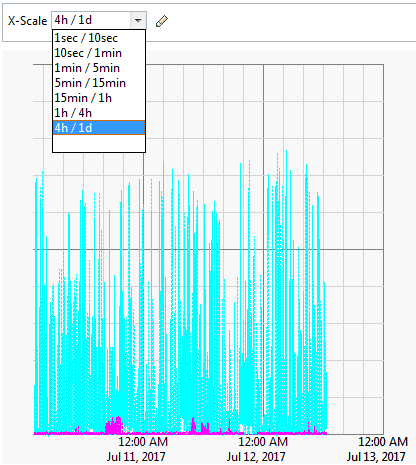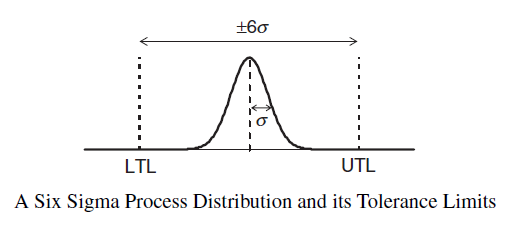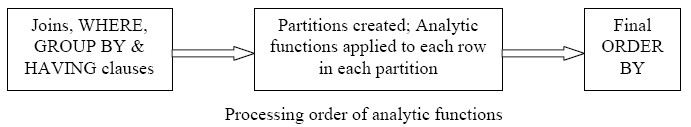# Distribution - (Mean|Average) (M| | )

The average is a measure of center that statisticians call the mean.

To calculate the mean, you add all numbers and divide the total by the number of numbers (N).

$$mean = \frac\sum_{i=1}^{i=N}{x_i}}{N}$$

The mean is not resistant.

The mean is such an important measure of center because it is the numerical “balancing point” of the data set.

The mean is representative of the entire sample, if you don't have any skew or outliers effect.

Averaging is not an additive operation. You can’t average an average.

A sample mean represents a single point in a sampling distribution and is then an estimator.

The Will Rogers phenomenon is obtained when moving an element from one set to another set raises the average values of both sets. See Will Rogers phenomenon

## Accuracy Statistic

### Standard Error

The standard error for the mean is estimated from:

under the assumption that the sample is random, normal and representative of the population.

$$\text{Standard Error (SE)} = \frac{\href{Standard Deviation}{\text{Standard Deviation (SD)}}}{\sqrt{\href{Sample Size}{\text{Sample Size (N)}}}}$$

The standard error increases then with an high variance (ie Standard deviation ) and a smallest sample size.

#### Example

Illustration of standard error calculation with R and the describe function (from the package psych)

require(psych)

describe(myData)
# se is the standard error for the mean

vars    n mean   sd median trimmed  mad   min  max range skew kurtosis   se
X1    1 1000 0.33 0.67   0.28    0.30 0.64 -0.81 1.85  2.66 0.44    -0.49 0.02
X2    2 1000 0.13 0.60   0.18    0.12 0.50 -1.18 1.77  2.94 0.17     0.31 0.02
y     3 1000 0.35 0.58   0.30    0.34 0.58 -0.75 2.04  2.79 0.42     0.18 0.02


# se = sd / sqrt(N)
descTable <- describe(myData)
descTable
myVariable.sd <- descTable[2,4]
myVariable.sd
myVariable.n <- descTable[2,2]
myVariable.n
myVariable.se <- descTable[2,4] / sqrt(descTable[2,2])
myVariable.se
myVariable.se == descTable[2,13]

TRUE



### t-statistic

The t-value statistic is some observed value minus an expected value relative to standard error.

$$\begin{array}{rrl} \text{t-value} & = & \frac{(\text{Observed} - \text{Expected})}{\href{Standard_Error}{\text{Standard Error}}} & \\ & = & \frac{(\href{Mean}{\text{Mean}} - 0)}{\href{Standard_Error}{\text{Standard Error}}} & \href{nhst}{\text{Playing the game of NHST the expected value is 0.}}\\ & = & \frac{\href{Mean}{\text{Mean}}}{\href{Standard_Error}{\text{Standard Error}}} & \\ \end{array}$$

This t-statistics is the base of the t-test.

???

## Documentation / Reference

Discover More(Prediction|Recommender System) - Collaborative filtering

Collaborative filtering is a method of making automatic predictions (filtering) the interests of a user by collecting preferences or taste information from many users (collaborating). But in general,...Application Metrics (Perfcounter | Performance Metrics | Operational data | Monitoring )

Application Metrics (Perfcounter | Performance Metrics | Operational data | Monitoring ) This section is about the collection and calculation of metrics in the monitoring context. These (counter|numbers)...Six Sigma (6s) is an approach to improve the performance of business process. where: sigma UTL = Upper Tolerance Limit. See LTL = Lower Tolerance Limit The 6s strategy was developed by Motorola,...Distribution - Measures of (center|central tendency) (Mean, Median, Mode)

A Measure of central tendency is a measure that describes the middle or center point of a distribution. A good measure of central tendency is representative of the distribution. The mean, the median and...Function - (Aggregate | Aggregation)

Aggregate functions return a single value calculated or selected from values that are in a aggregation relationship (ie a set) This values are also known as summary because they try to summarize...How to use the Aggregate / Window Functions (sum, avg, ) in Sqlite ?

... The aggregate / window function in Sqlite. Sqlite supports the following aggregate /window function : SUM (total) AVG Max Min Rank row_number more see the Specification...Performance - Utilization

A percent over a time interval. eg, “one disk is running at 90% utilization”. mean Utilization is also known as loadQuantile - (Median|Middle)

The median is a measure of center. The middle number of a set of data is the median. This measure is resistant. The median is a 50th percentile (or “middle” quartile). Half of the data is below the...R - Mean

This function calculate the mean. You can find it also in the summary statistics functionsSQL Function - Window Aggregate (Analytics function)

Windowing functions (known also as analytics) allow to compute: cumulative, moving, and aggregates. They are distinguished from ordinary SQL functions by the presence of an OVER clause. With...